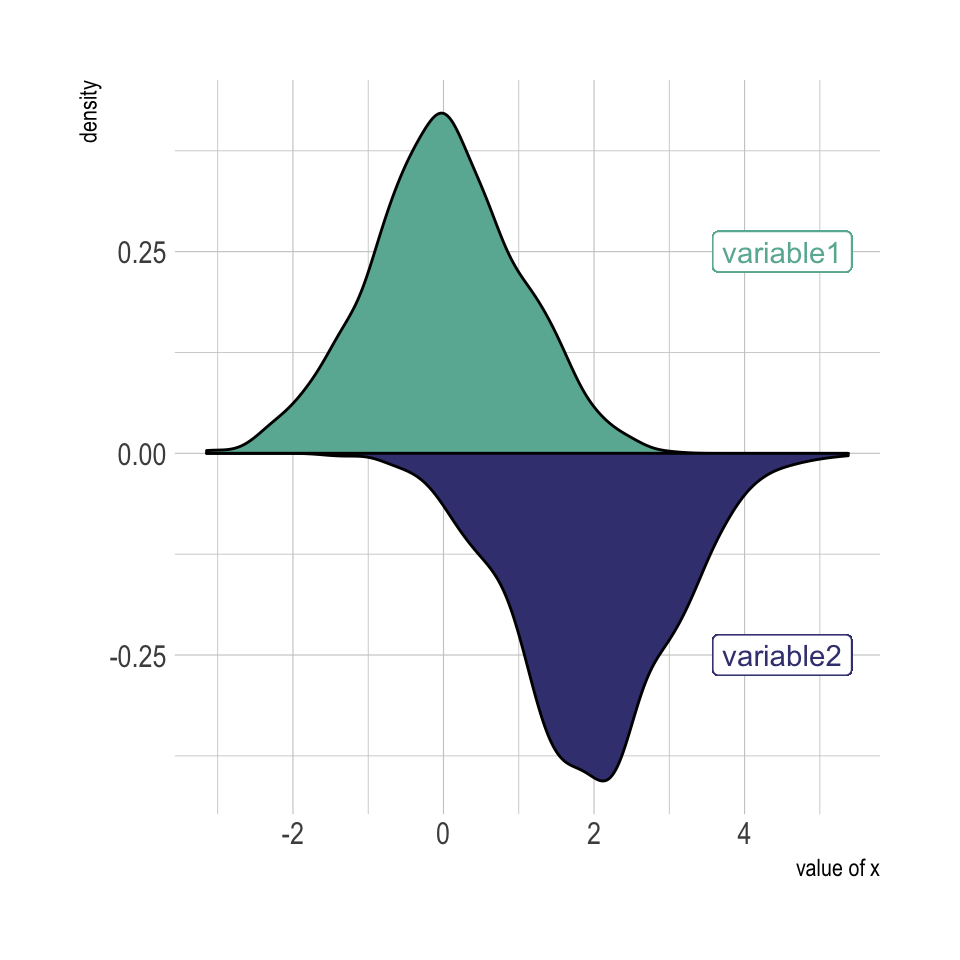# Mirror density chart with ggplot2

A density plot is a representation of the distribution of a numeric variable. Comparing the distribution of 2 variables is a common challenge that can be tackled with the mirror density chart: 2 density charts are put face to face what allows to efficiently compare them. Here is how to build it with ggplot2 library.

# Density with `geom_density`

A density chart is built thanks to the `geom_density` geom of ggplot2 (see a basic example). It is possible to plot this density upside down by specifying `y = -..density..`. It is advised to use `geom_label` to indicate variable names.

``````# Libraries
library(ggplot2)
library(hrbrthemes)

# Dummy data
data <- data.frame(
var1 = rnorm(1000),
var2 = rnorm(1000, mean=2)
)

# Chart
p <- ggplot(data, aes(x=x) ) +
# Top
geom_density( aes(x = var1, y = ..density..), fill="#69b3a2" ) +
geom_label( aes(x=4.5, y=0.25, label="variable1"), color="#69b3a2") +
# Bottom
geom_density( aes(x = var2, y = -..density..), fill= "#404080") +
geom_label( aes(x=4.5, y=-0.25, label="variable2"), color="#404080") +
theme_ipsum() +
xlab("value of x")

#p``````# Histogram with `geom_histogram`

Of course it is possible to apply exactly the same technique using `geom_histogram` instead of `geom_density` to get a mirror histogram:

``````# Chart
p <- ggplot(data, aes(x=x) ) +
geom_histogram( aes(x = var1, y = ..density..), fill="#69b3a2" ) +
geom_label( aes(x=4.5, y=0.25, label="variable1"), color="#69b3a2") +
geom_histogram( aes(x = var2, y = -..density..), fill= "#404080") +
geom_label( aes(x=4.5, y=-0.25, label="variable2"), color="#404080") +
theme_ipsum() +
xlab("value of x")

#p``````Related chart types

## Contact

This document is a work by Yan Holtz. Any feedback is highly encouraged. You can fill an issue on Github, drop me a message on Twitter, or send an email pasting yan.holtz.data with gmail.com.